# Consecutive Angles

Consecutive Angles

Renee was observing the streets through her room's window. She noticed that the horizontal bars in the window column were parallel and crossed over by a vertical bar. She wondered if she could relate the angles formed by the bars to the concept of consecutive angles.Let us see if we can relate to it.

In this section, we will explore the consecutive angles, consecutive interior angles, consecutive exterior angles, consecutive angles in a parallelogram, as well as consecutive interior angles theorem using real-life examples.

Check-out the interactive simulation to know more about the lesson and try your hand at solving a few interesting practice questions on consecutive angles at the end of the page.

## Lesson Plan

 1 What Do You Mean by Consecutive Angles? 2 Thinking Out of the Box! 3 Solved Examples on Consecutive Angles 4 Challenging Questions on Consecutive Angles 5 Interactive Questions on Consecutive Angles

## What Do You Mean by Consecutive Angles?

When two parallel lines are crossed over by a transversal, they are divided into two sections 1 and 2, as shown in the figure below.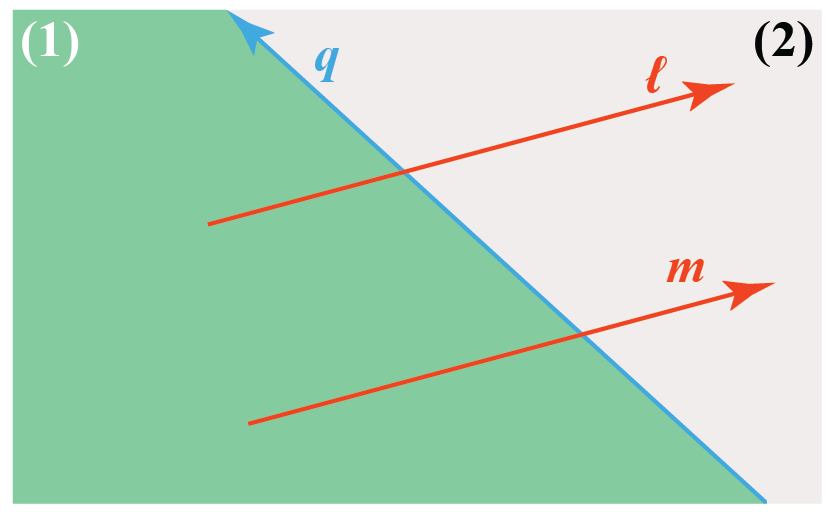Here 'l' and 'm' represent the parallel lines and 'q' represents the transversal.

The pair of supplementary angles on any one of the sections of the transversal forms consecutive angles.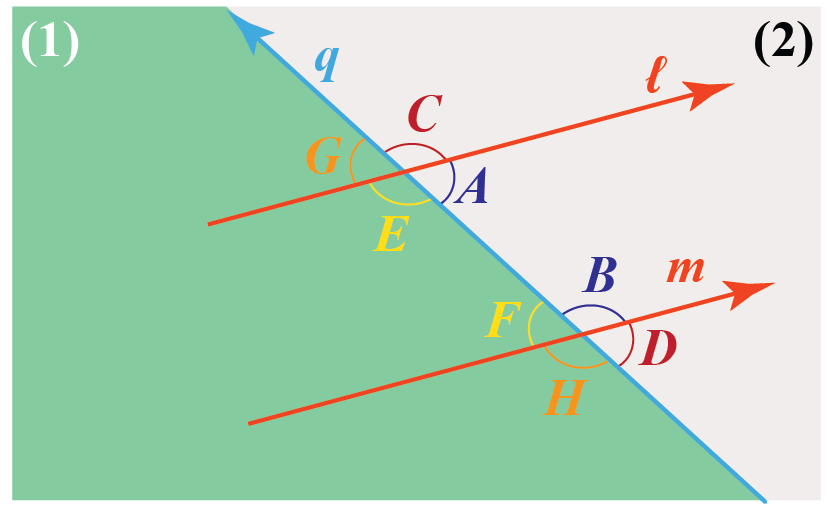The pair of consecutive angles for section 1 in the above figure can be named as $$(\angle \text A, \angle \text B)$$ and$$(\angle \text C, \angle \text D)$$.

Similarly for the section 2 are $$(\angle \text E, \angle \text F)$$ and $$(\angle \text G, \angle \text H)$$ are the pair of consecutive angles.

## What Are the Types of Consecutive Angles?

Consecutive angles can be divided into two parts according to their position with relation to parallel lines and the transversal. i.e.,

1. Consecutive interior angles
2. Consecutive exterior angles

Consecutive interior angles:

These angles lie on the inside of the two parallel lines and on the same side of the transversal.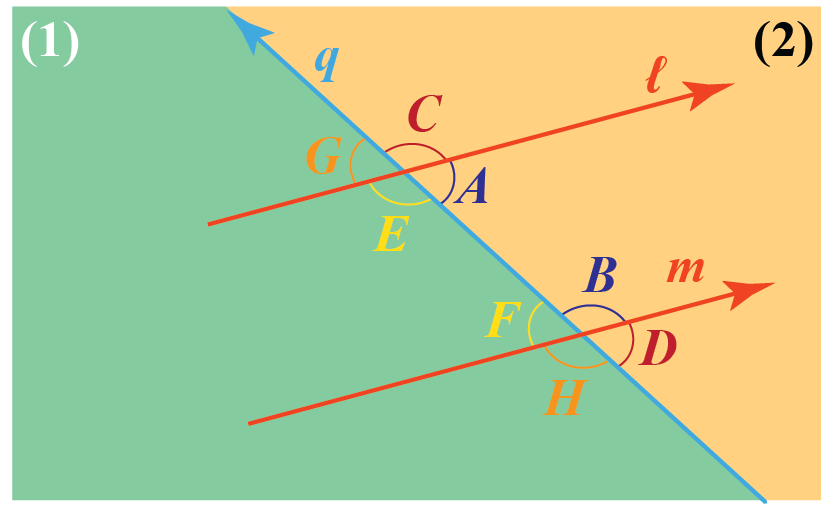The pair of consecutive interior angles for the two sections in the above figure can be named as $$(\angle \text A, \angle \text B)$$ and $$(\angle \text E, \angle \text F)$$.

Note: Consecutive interior angles are supplementary angles, i.e., they add up to 180$$^\circ$$.

This can be proved by the consecutive interior angles theorem which states that "If a transversal intersects two parallel lines, each pair of consecutive interior angles are supplementary (their sum is 180$$^\circ$$)."

The proof of the above-stated theorem is given below.

## Consecutive Interior Angles Theorem - Proof

Refer to the following figure once again: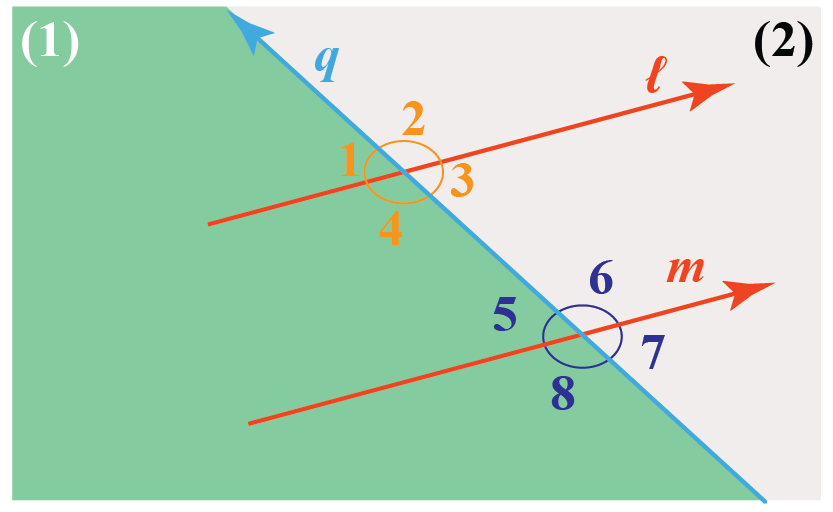We have:

\begin{align} \angle 4& = \angle 8 \;\;\;\text{ (corresponding angles)} \\[0.3cm]\angle 5 + \angle8& = 180^\circ \;\text{ (linear pair)}\end{align}

From the above two equations, $\angle 4 + \angle5 = 180^\circ$.

Similarly, we can show that $\angle 6 + \angle 3 = 180^\circ$.

Consecutive exterior angles:

These angles lie on the outside of the two parallel lines and on the same side of the transversal.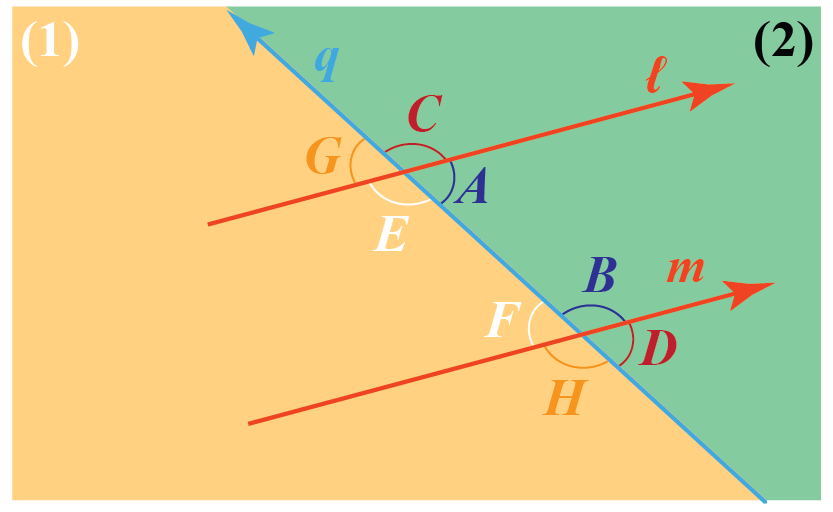The pair of consecutive exterior angles for the two sections in the above figure can be named as $$(\angle \text C, \angle \text D)$$ and $$(\angle \text G, \angle \text H)$$.

Example

Interior consecutive angles in a parallelogram can be seen in the following image: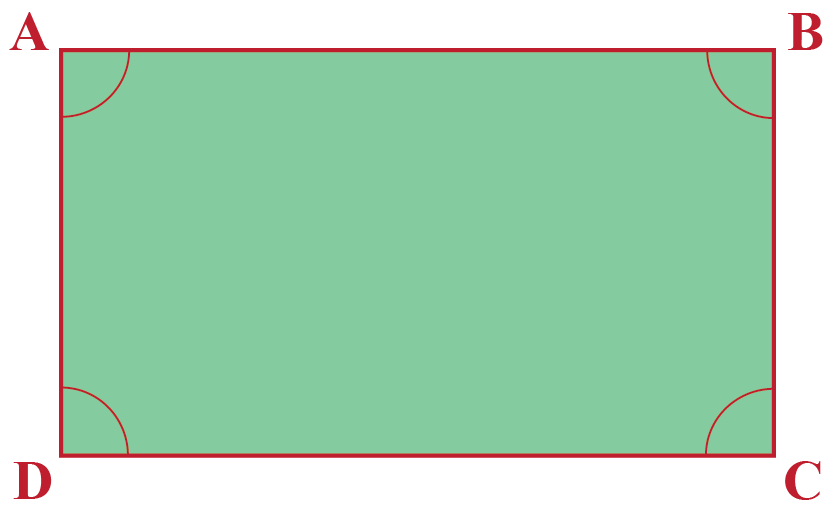The pair of consecutive angles for the parallelogram can be named as $$(\angle \text A, \angle \text D)$$, $$(\angle \text A, \angle \text B)$$, $$(\angle \text C, \angle \text D)$$, and $$(\angle \text C, \angle \text B)$$.Think Tank
• Can you figure out whether the converse of the consecutive interior angle theorem is true or not?

## Solved Examples

 Example 1

Two consecutive angles of a parallelogram are in the ratio of 1:8. Can you find out the value of the smaller angle?

Solution

Let the smaller angle be 'x', the bigger angle be '8x'.

Since,$$\angle \text{ A and }\angle \text B$$ are consecutive angles,

$$\therefore \angle \text A + \angle \text B = 180^\circ$$

Hence,\begin{align*} \text x + 8\text x &= 180^\circ \\ 9\text x &= 180^\circ \\ \text x &= 20^\circ \end{align*}

 $$\therefore$$ The smaller angle is 20$$^\circ$$.
 Example 2

Sean wants to find if the two lines 'p' and 'q' shown in the figure are parallel or not?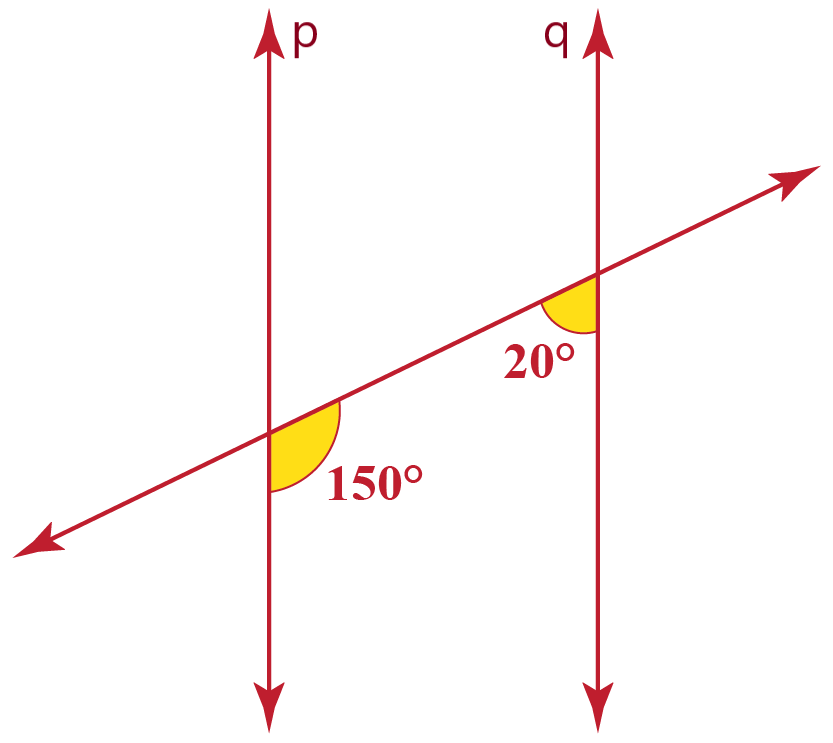Solution

In the given figure, both the shown angles are interior angles.

If p and q are the parallel lines, then they must follow the consecutive interior angle theorem, which means the angles must be supplementary.

But $150^\circ + 20^\circ ≠ 180^\circ$

This shows that they are not supplementary angles.

 $$\therefore$$ p and q are not parallel
 Example 3

Mark was given a parallelogram with one of the angles as $$\angle W = (\text a - 10)^\circ$$, where a = 70°.  Can you help Mark find out the value of  $$\angle Y$$?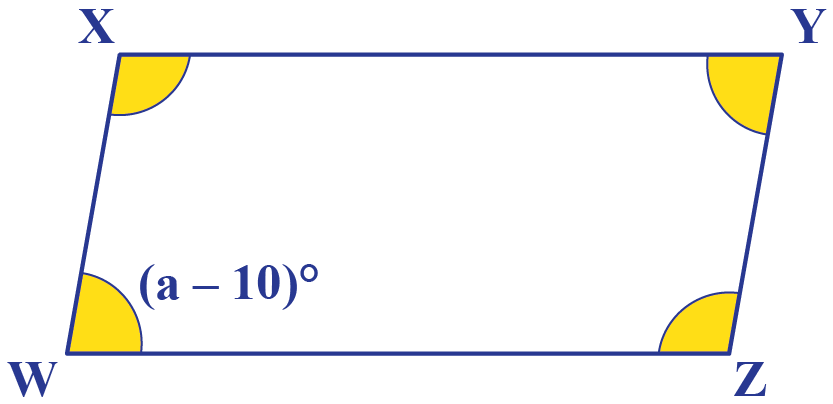Solution

Given,  $$\angle W = (\text a - 10)^\circ$$

Since $$\angle W$$and  $$\angle X$$ are consecutive angles, we can write-

$\angle W + \angle X = 180$  On substituting the given values, we get-\begin{align*} (\text a - 10) + \angle X &= 180 \\ 70 - 10 + \angle X &= 180 \\ \angle X &= 180 - 60 \\ \angle X &= 120 \end{align*}

Similarly, $$\angle X$$and  $$\angle Y$$ are consecutive angles, hence we can write-

$\angle X + \angle Y = 180$ On substituting the values, we get-  \begin{align*} 120+ \angle Y &= 180 \\ \angle Y &= 180 - 120 \\ \angle Y &= 60 \end{align*}

An interesting result is seen here. i.e., $$\angle \text W = \angle \text Y$$.

 $$\therefore \angle \text W = 60^\circ$$Challenging Question
• Euclid's fifth postulate states that if a straight line crossing two straight lines makes the interior angles on the same side less than two right angles, the two straight lines if extended indefinitely, meet on that side on which the angles are less than the two right angles.

Can you compare this postulate with the consecutive interior angle theorem?

## Interactive Questions

Here are a few activities for you to practice. Select/Type your answer and click the "Check Answer" button to see the result.

## Let's Summarize

This mini-lesson targeted the fascinating concept of consecutive angles. The math journey around the consecutive angles starts with what a student already knows, and goes on to creatively crafting a fresh concept in the young minds. Done in a way that not only it is relatable and easy to grasp, but also will stay with them forever. Here lies the magic with Cuemath.

## About Cuemath

At Cuemath, our team of math experts is dedicated to making learning fun for our favorite readers, the students!

Through an interactive and engaging learning-teaching-learning approach, the teachers explore all angles of a topic.

Be it worksheets, online classes, doubt sessions, or any other form of relation, it’s the logical thinking and smart learning approach that we, at Cuemath, believe in.

## 1. What are consecutive interior angles?

When two parallel lines are crossed by a transversal, then the pairs of angles on one side of the transversal but inside the two lines are called consecutive interior angles.

## 2. What are consecutive exterior angles?

When two parallel lines are crossed by a transversal, then the pairs of angles on one side of the transversal but outside the two lines are called consecutive exterior angles.

More Important Topics
Numbers
Algebra
Geometry
Measurement
Money
Data
Trigonometry
Calculus